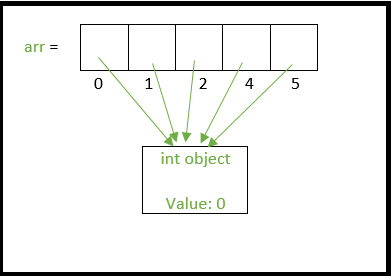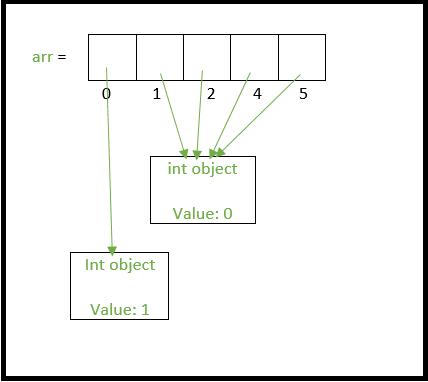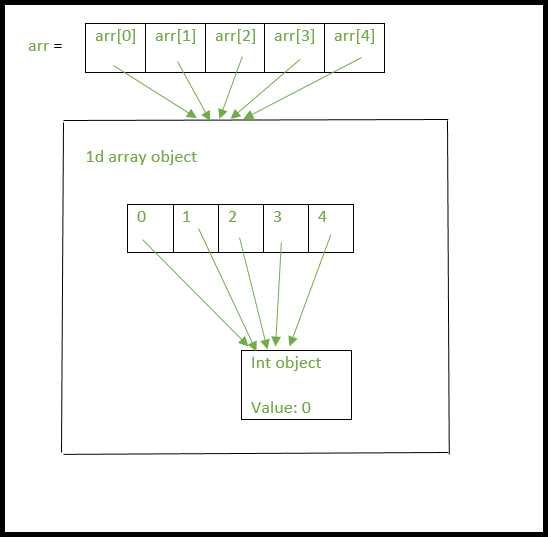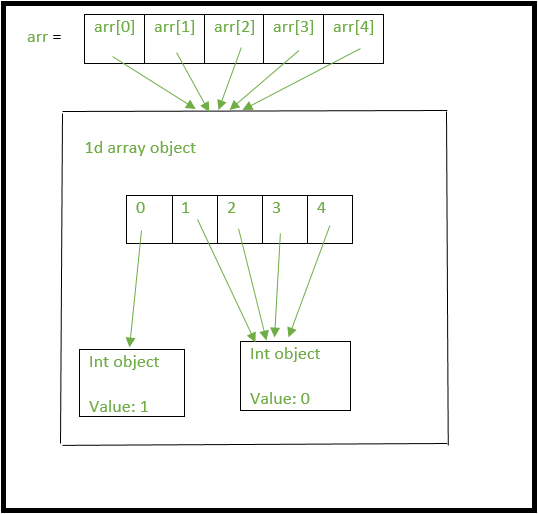# Python | Using 2D arrays/lists the right way

• Difficulty Level : Easy
• Last Updated : 21 Jun, 2022

Python provides many ways to create 2-dimensional lists/arrays. However one must know the differences between these ways because they can create complications in code that can be very difficult to trace out. Lets start by looking at common ways of creating 1d array of size N initialized with 0s.Method 1a

## Python3

 `# First method to create a 1 D array ` `N ``=` `5` `arr ``=` `[``0``]``*``N ` `print``(arr) `

Output:

`[0, 0, 0, 0, 0]`

Method 1b

## Python3

 `# Second method to create a 1 D array ` `N ``=` `5` `arr ``=` `[``0` `for` `i ``in` `range``(N)] ` `print``(arr) `

Output:

`[0, 0, 0, 0, 0]`

Extending the above we can define 2-dimensional arrays in the following ways.
Method 2a

## Python3

 `# Using above first method to create a  ` `# 2D array ` `rows, cols ``=` `(``5``, ``5``) ` `arr ``=` `[[``0``]``*``cols]``*``rows ` `print``(arr) `

Output:

`[[0, 0, 0, 0, 0], [0, 0, 0, 0, 0], [0, 0, 0, 0, 0], [0, 0, 0, 0, 0], [0, 0, 0, 0, 0]]`

Method 2b

## Python3

 `# Using above second method to create a  ` `# 2D array ` `rows, cols ``=` `(``5``, ``5``) ` `arr ``=` `[[``0` `for` `i ``in` `range``(cols)] ``for` `j ``in` `range``(rows)] ` `print``(arr) `

Output:

`[[0, 0, 0, 0, 0], [0, 0, 0, 0, 0], [0, 0, 0, 0, 0], [0, 0, 0, 0, 0], [0, 0, 0, 0, 0]]`

Method 2c

## Python3

 `# Using above second method to create a  ` `# 2D array ` `rows, cols ``=` `(``5``, ``5``) ` `arr``=``[] ` `for` `i ``in` `range``(rows): ` `    ``col ``=` `[] ` `    ``for` `j ``in` `range``(cols): ` `        ``col.append(``0``) ` `    ``arr.append(col) ` `print``(arr) `

Output:

`[[0, 0, 0, 0, 0], [0, 0, 0, 0, 0], [0, 0, 0, 0, 0], [0, 0, 0, 0, 0], [0, 0, 0, 0, 0]]`

Both the ways give seemingly same output as of now. Lets change one of the elements in the array of method 2a and method 2b.

## Python3

 `# Python 3 program to demonstrate working  ` `# of method 1 and method 2. ` ` `  `rows, cols ``=` `(``5``, ``5``) ` ` `  `# method 2a ` `arr ``=` `[[``0``]``*``cols]``*``rows ` ` `  `# lets change the first element of the  ` `# first row to 1 and print the array ` `arr[``0``][``0``] ``=` `1` ` `  `for` `row ``in` `arr: ` `    ``print``(row) ` `# outputs the following ` `#[1, 0, 0, 0, 0] ` `#[1, 0, 0, 0, 0] ` `#[1, 0, 0, 0, 0] ` `#[1, 0, 0, 0, 0] ` `#[1, 0, 0, 0, 0] ` ` `  `# method 2b ` `arr ``=` `[[``0` `for` `i ``in` `range``(cols)] ``for` `j ``in` `range``(rows)] ` ` `  `# again in this new array lets change ` `# the first element of the first row  ` `# to 1 and print the array ` `arr[``0``][``0``] ``=` `1` `for` `row ``in` `arr: ` `    ``print``(row) ` ` `  `# outputs the following as expected ` `#[1, 0, 0, 0, 0] ` `#[0, 0, 0, 0, 0] ` `#[0, 0, 0, 0, 0] ` `#[0, 0, 0, 0, 0] ` `#[0, 0, 0, 0, 0] `

Output:

```[1, 0, 0, 0, 0]
[1, 0, 0, 0, 0]
[1, 0, 0, 0, 0]
[1, 0, 0, 0, 0]
[1, 0, 0, 0, 0]
[1, 0, 0, 0, 0]
[0, 0, 0, 0, 0]
[0, 0, 0, 0, 0]
[0, 0, 0, 0, 0]
[0, 0, 0, 0, 0]```

We expect only the first element of first row to change to 1 but the first element of every row gets changed to 1 in method 2a. This peculiar functioning is because Python uses shallow lists which we will try to understand.
In method 1a, Python doesn’t create 5 integer objects but creates only one integer object and all the indices of the array arr point to the same int object as shown.If we assign the 0th index to a another integer say 1, then a new integer object is created with the value of 1 and then the 0th index now points to this new int object as shown belowSimilarly, when we create a 2d array as “arr = [*cols]*rows” we are essentially the extending the above analogy.
1. Only one integer object is created.
2. A single 1d list is created and all its indices point to the same int object in point 1.
3. Now, arr, arr, arr …. arr[n-1] all point to the same list object above in point 2.
The above setup can be visualized in the image below.Now lets change the first element in first row of “arr” as
arr = 1
=> arr points to the single list object we created we above.(Remember arr, arr …arr[n-1] all point to the same list object too)
=> The assignment of arr will create a new int object with the value 1 and arr will now point to this new int object.(and so will arr, arr …arr[n-1])
This can be clearly seen in the below image.So when 2d arrays are created like this, changing values at a certain row will effect all the rows since there is essentially only one integer object and only one list object being referenced by the all the rows of the array.
As you would expect, tracing out errors caused by such usage of shallow lists is difficult. Hence the better way to declare a 2d array is

## Python3

 `rows, cols ``=` `(``5``, ``5``) ` `arr ``=` `[[``0` `for` `i ``in` `range``(cols)] ``for` `j ``in` `range``(rows)] `

Output:

` `

This method creates 5 separate list objects unlike method 2a. One way to check this is using the ‘is’ operator which checks if the two operands refer to the same object.

## Python3

 `rows, cols ``=` `(``5``, ``5``) ` `# method 2b ` `arr ``=` `[[``0` `for` `i ``in` `range``(cols)] ``for` `j ``in` `range``(rows)] ` ` `  `# check if arr and arr refer to ` `# the same object ` `print``(arr[``0``] ``is` `arr[``1``]) ``# prints False ` ` `  `# method 2a ` `arr ``=` `[[``0``]``*``cols]``*``rows ` ` `  `# check if arr and arr refer to  ` `# the same object ` `# prints True because there is only one ` `# list object being created. ` `print``(arr[``0``] ``is` `arr[``1``]) `

Output:

```False
True```

My Personal Notes arrow_drop_up
Recommended Articles
Page :